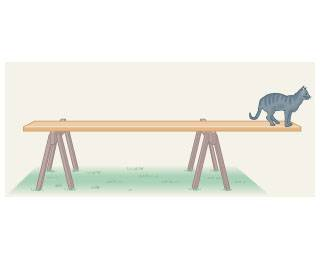# Cat on a plank torque problem

Andrews989

## Homework Statement

A cat walks along a uniform plank that is 4.20 long and has a mass of 5.00 . The plank is supported by two sawhorses, one 0.440 from the left end of the board and the other 1.50 from its right end.

If the cat has a mass of 3.3 , how close to the right end of the two-by-four can it walk before the board begins to tip?## Homework Equations

Net force = 0
Net torque = 0

F=ma

Torque = r * F * sin(theta)

## The Attempt at a Solution

Since the board is just beginning to tip, there is no weight on the left sawhorse.

So the right side the torque is equal to the force (mass * g) times the distance which is the unkown?

Force = 3.3*9.81 = 32.37 N

Torque = 32.37 N * d?

I'm stuck....

## Answers and Replies

Mentor
So far, so good. You found an expression for the clockwise torque due to the cat's weight. What's providing the counter-clockwise torque?

Andrews989
So far, so good. You found an expression for the clockwise torque due to the cat's weight. What's providing the counter-clockwise torque?

Mass of the board. Which you count the mass from the center of gravity.

So, the plank is 4.2m. The center is 2.1m. The axis of rotation is 1.5m from the right. Making the plank .6 meters from the axis of rotation (to the left).

The the torque of the plank is .6m times 5kg * 9.81 = 29.3.

So the torque on the left has to equal the torque on the right?

29.3 = 32.37 N * d

d = .91 meters?

It's online homework and that is wrong... so something is wrong....

Mentor
You found the cat's distance from the pivot. How far is that from the end?

Andrews989
You found the cat's distance from the pivot. How far is that from the end?

Oh. Didn't think about that. So 1.5 - .91 = .59 meters.....which is the correct answer. Thanks!